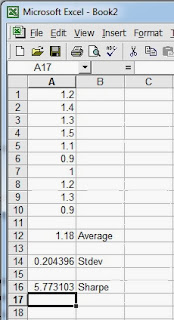## Pages

### Sharpe Ratio for Betting

David Edelman's The Compleat Horseplayer gives a betting equivalent for the Sharpe Ratio (pages 27 & 28). The Sharpe Ratio (aka Information Ratio) is used as a measure of effectiveness for a strategy.

In finance, the Sharpe Ratio is defined "as the excess return (or risk premium) per unit of deviation in an investment asset or a trading strategy, typically referred to as risk (and is a deviation risk measure), named after William Forsyth Sharpe." The ratio measures how well the return of an asset compensates the investor for the risk taken.

In sports betting the Sharpe Ratio is a measure of how much a trader is rewarded for any particular system they devise.The ratio is given by the excess returns divided by standard deviation (aka volatility) and can be computed in Excel with the formula

=average(a1:a10) / stdev(a1:a10)

where a1:10 is your array of returns from any single strategy.

To the right you can see a sample of returns for which I have created a Sharpe Ratio. Play around with the returns (percentage or absolute) in cells A1 to A10. Put in some negative values to denote losses and also widely differing positive and negative returns to get a feel for what the Sharpe Ratio measures.

For example, you only have to change one of the values to -1.0 and the standard deviation will increase thus dramatically lowering the ratio.

In finance a good ratio is regarded as being greater than 2. In other words, your average return for any trading strategy should be twice that of the standard deviation of the returns. A Sharpe Ratio of 1 means that the return is equal to the risk being taken and less than 1 means that more risk is being taken than the reward in return. The higher the Sharpe Ratio then the lower the deviation of returns compared with the average. In other words, no sudden big losses that can wipe out your bankroll. Slow and steady wins the race.

For sports trading I have tried to calculate a Sharpe Ratio after every trade but that produces very low figures. Instead, I calculate a figure for total yield at the end of the day and produce a Sharpe Ratio based on daily yields. Generally, the longer the period the larger the ratio. You could calculate a weekly or monthly yield and base your ratio on that. However, if your trading models are short lived then the daily ratio is probably the best.

I use Sharpe Ratio as one of many fitness measures when developing new trading models. See GA Optimisation of Entries and Exits.